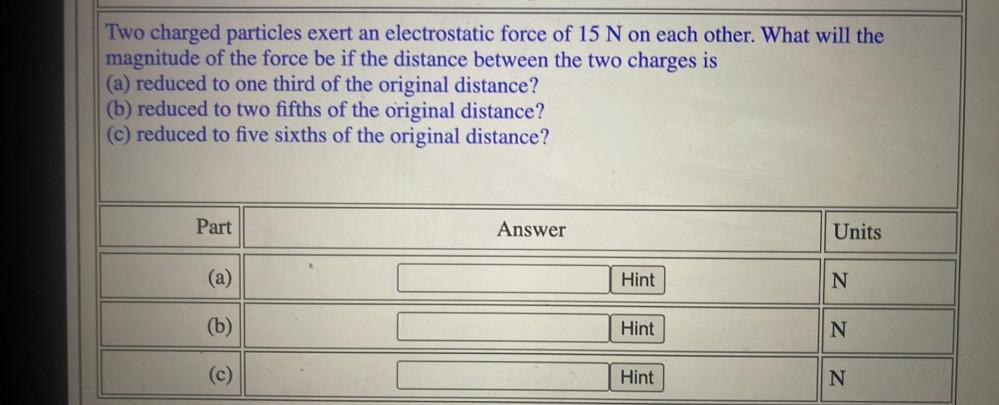Question:

# Two charged particles exert an electrostatic force of 15 N on each other. What will the magnitude of the force be if the distancTwo charged particles exert an electrostatic force of 15 N on each other. What will the magnitude of the force be if the distance between the two charges is (a) reduced to one third of the original distance? (b) reduced to two fifths of the original distance? (c) reduced to five sixths of the original distance? Part Answer Units (a) Hint N (b) Hint N N N (c) Hint